## 17 Jul 2016

### Frege, “Function and Concept”, summary

[Search Blog Here. Index-tags are found on the bottom of the left column.]

[Gottlob Frege, Entry Directory]

[The following, except for my bracketed commentary and off-set quotations, is summary. Boldface is my addition. Please forgive my typos and other errors, as I did not complete the proofreading yet. Note also the notation convention used in the text here for multiplication. It will appear as a decimal point between numbers or variables, but it means multiplication.]

Summary of

Gottlob Frege

“Function and Concept”

(Geach transl.)

Brief Summary:

The notion of a function found its development and expression in the history of mathematics, becoming explicitly examined with the invention of the calculus. In mathematics, the function is understood as a formula containing the variable x. Frege advances the notion of function and further develops it as a logical concept by defining it as having a very specific structure. A function’s structure has two main parts, an argument and a function. The argument part does not belong to the function, because it must be understood as variable, and thus it can be regarded as an empty placeholder belonging with the function. A function is unsaturated when the argument is not specified as one object or another, as when a variable symbol is used in the argument place, and it is saturated when one specific argument or another is given in the argument place. We say that two functions equal one another when they have the same range of values for the same arguments. To express the generality (or variability) of functions themselves we will use the letters f and F; so: ‘f(x),’ ‘F(x),’ where ‘x’ replaces the argument. Now suppose we have the function x2 = 1. And suppose further that we replace the x with the series of values – 1, o, 1, 2. Only in the first and third cases is the resulting equation true. The value of a function is a truth value. The value of the first and third is the True, and the value of the second and fourth is the False. Here we must distinguish the sense (Sinn) from the reference (Bedeutung) of the expression. The sense is the thought expressed in the expression, and the reference is the specific object or value being represented. So functions, when their arguments are supplied, may have different references, that is, different numerical values, but their senses can be the same, namely, being either true or false. Thus (22 = 4) = (2 > 1) is a correct formulation, when they are instantiations of the functions x2 = 4 and x > 1, because they both stand for the true and thus equal one another with regard to their senses. A concept can be understood as a function in the sense that the function element is like the conceptual predication of the argument. The “capital of England” is an unsaturated function. When its argument is London, it is true. So the proposition “London is the capital of England” has as its “thought,” reference, or sense the True.

Summary

Frege will discuss some ideas that expand upon what he says in his Begriffsschrift (Frege 21).

He begins with the notion of function as it is understood in mathematics, and then secondly he will look at how the notion of function has widened beyond its mathematical sense (21). The sort of functions he begins with just have a single argument. [I am not sure what he says in the following, so I will quote it. He might be saying that scientific descriptions of laws were made possible by the way the function came to be used with the invention of the calculus. By going back to this time in the history of mathematics, we learn that the meaning of function is a mathematical expression containing a variable.]

The first place where a scientific expression appears with a clear-cut reference is where it is required for the statement p. 2] of a law. This case arose, as regards the function, upon the discovery of higher Analysis. Here for the first time it was a matter of setting forth laws holding for functions in general. So we must go back to the time when higher Analysis was discovered, if we want to know what the word ‘function’ was originally taken to mean. The answer that we are likely to get to this question is: ‘A function of x was taken to be a mathematical expression containing x, a formula containing the letter x.’ Thus, e.g., the expression

2.x3 + x

would be a function of x, and

2.23 + 2

would be a function of 2.

(Frege 21)

[I am not quite sure I follow. In the first case, it would seem to be a function of x, because the value of the expression will vary depending on what value you give to the x variable. In the second case, there is no variance in the value, because there is no variable. It therefore would not seem to satisfy the definition he gave it, which required the variable x. I suppose then that what is going on is there was a substitution of x by 2, and although normally 2 would not be considered a function in that equation, it does in this case, since it was obtained by modifying a given function. In that case, I think the dot in 2.23 would have to mean multiplication (rather than decimal); for otherwise I do not understand the substitution. In a German version, the dot is elevated a little. Compare: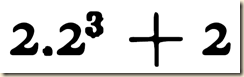(Frege “Function and Concept”)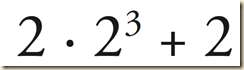(Frege “Funktion und Begriff”)

] Frege says that this mathematical definition of a function is not satisfactory, because it does not make a distinction between form and content, and between sign and thing signified. [I do not follow everything here. It seems the idea is that in the equation above, the form or sign is 2.23 + 2 and the content or thing signified is 16. For this reason, one content or signified value can take many signs. Those signs should not bee seen as having identities independent of one another. Frege offers a few other examples, with his point always being that it does not matter how the value is formulated, it always has the same value. He emphasizes that even though these other formulations might “correspond to different conceptions and aspects,” they nevertheless always are expressions of the same thing. What is important is how all this relates to the definition of the function, and here it is less obvious to me what his point is. He might be saying that 2.x3 + x is a function, but the reason for this is not that it represents some number in an indefinite way. You will have to read the last few sentences of this paragraph to discern his point. But he might be saying that if the expression is thought to be variable depending on the x value, then it is a function. But if it is thought to have some certain value only we do not designate it at the moment, then we are not thinking of it as a function.]

This answer cannot satisfy us, for here no distinction is made between form and content, sign and thing {21|22} signified; a mistake, admittedly, that is very often met with in mathematical works, even those of celebrated authors. I have already pointed out on a previous occasion the defects of the current formal theories in arithmetic. We there have talk about signs that neither have nor are meant to have any content, but nevertheless properties are ascribed to them which are unintelligible except as belonging to the content of a sign. So also here; p. 3] a mere expression, the form for a content, cannot be the heart of the matter; only the content itself can be that. Now what is the content of ‘2.23 + 2’? What does it stand for? The same thing as ‘18’ or ‘3.6.’ What is expressed in the equation ‘2.23 + 2 = 18’ is that the right-hand complex of signs has the same reference as the left-hand one, I must here combat the view that, e.g., 2 + 5 and 3 + 4 are equal but not the same. This view is grounded in the same confusion of form and content, sign and thing signified. It is as though one wanted to regard the sweet-smelling violet as differing from Viola odorata because the names sound different. Difference of sign cannot by itself be a sufficient ground for difference of the thing signified. The only reason why in our case the matter is less obvious is that the reference of the numeral 7 is not anything perceptible to the senses. There is at present a very widespread tendency not to recognize as an object anything that cannot be perceived by means of the senses; this leads here to numerals’ being taken to be numbers, the proper objects of our discussion; and then, I admit, 7 and 2 + 5 would indeed be different. But such a conception p. 4] is untenable, for we cannot speak of any arithmetical properties of numbers whatsoever without going back to what the signs stand for. For example, the property belonging to 1, of being the result of multiplying itself by itself, would be a mere myth; for no microscopical or chemical investigation, however far it was carried, could ever detect this property in the possession of the innocent character that we call a figure one. Perhaps there is talk of a definition; but no definition is creative in the sense of being able to endow a thing with properties that {22|23} it has not already got – apart from the one property of expressing and signifying something in virtue of the definition. The characters we call numerals have, on the other hand, physical and chemical properties depending on the writing material. One could imagine the introduction some day of quite new numerals, just as, e.g., the Arabic numerals superseded the Roman. Nobody is seriously going to suppose that in this way we should get quite new numbers, quite new arithmetical objects, with properties still to be investigated. Thus we must distinguish between numerals and what they stand for; and if so, we shall have to recognize that the expressions ‘2,’ ‘1 + 1,’ ‘3 - 1,’ ‘6 :3’ p. 5] stand for the same thing, for it is quite inconceivable where the difference between them could lie. Perhaps you say: 1 + 1 is a sum, but 6:3 is a quotient. But what is 6:3? The number that when multiplied by 3 gives the result 6. We say ‘the number,’ not ‘a number’; by using the definite article, we indicate that there is only a single number. Now we have:

(1 + 1) + (1 + 1) + (1 + 1) = 6,

and thus (1 + 1) is the very number that was designated as (6:3). The different expressions correspond to different conceptions and aspects, but nevertheless always to the same thing. Otherwise the equation x2 = 4 would not just have the roots 2 and –2, but also the root (1 + 1) and countless others, all of them different, even if they resembled one another in a certain respect. By recognizing only two real roots, we are rejecting the view that the sign of equality does not stand for complete coincidence but only for partial agreement. If we adhere to this truth, we see that the expressions:

‘2.13 + 1,’

‘2.23 + 2,’

‘2.43 + 4,’

stand for numbers, viz. 3, 18, 132. So if the function were really the reference of a mathematical expression, it would just be a number; and nothing new would have been gained for arithmetic [by speaking of functions]. Admittedly, people who use the word {23|24} p. 6] ‘function’ ordinarily have in mind expressions in which a number is just indicated indefinitely by the letter x, e.g. ‘2.x3 + x’ ; but that makes no difference; for this expression likewise just indicates a number indefinitely, and it makes no essential difference whether I write it down or just write down ‘x.’

(Frege 21-24)

But this notion of the indefinite value is on track, because we need the concept of x indicating a value indefinitely. We often call x the argument, and we see the same function instantiated in each of these cases:

‘2.13 + 1,’

‘2.43 + 4,’

‘2.53 + 5,’

only in each case there are different arguments, namely, 1, 4, and 5 respectively. Thus we see that what is in common among these expressions is the way the x functions. We could also write it as

‘2.(   )3 +(   ).’

(Frege 24)

Frege will show that the argument “does not belong with the function, but goes together with the function to make up a complete whole; for the function by itself must be called incomplete, in need of supplementation, or ‘unsaturated’ ” (Frege 24).  [It is a little unclear what he means with the argument not “belonging with” the function but that it still “goes together with” it. The idea seems to be the following, if I had to guess. One conception of the function would say that the argument is a part of it, and therefore that the function cannot exist without the argument. Frege’s conception however considers the function as something that can exist without an argument. In that case, I would think that in when it exists without an argument, it would still (at least conventionally) take a variable like x in the example above. Otherwise, we would not know where the argument would be placed were a substitution made.] So we acknowledge that ‘2.13 + 1’ and ‘2.23 + 2’ have different values, that is different contents. However, we see them both expressing the same function, namely 2.x3 + x. Thus although ‘2.13 + 1’ and ‘4 – 1’ have the same values, we do not regard them as expressing the same function. [The next point I do not follow so well. He seems to be saying that people erroneously might think that the what is essential to a function is the form that it takes. For, functions often take a form where its parts can be split up somehow. I am not sure how, but perhaps the parts are the variable parts and the non-variable parts. And perhaps Frege is saying that the same function can be expressed in different ways, so long as it implies the same set of possible values. I am guessing, so let me quote:] “Moreover, we now see how people are easily led to regard the form of the expression as what is essential to the function. We recognize the function in the expression by imagining the latter, as split up, and the possibility of thus splitting it up is suggested by its structure” (Frege 24).

Frege distinguishes the two parts of the function as being “the sign of the argument” and the “expression of the function”. He says they are dissimilar, because the argument is a number, which is whole and complete in itself, while the function is not a whole and complete number. [I do not quite understand his illustration of a point dividing a line into two segments. The basic idea seems to be that if we think of a line terminating  at some point that divides it, it is like the argument, which is likewise determinate. But without such a dividing point, the line is not complete and entire. Let me quote as I cannot give a good explanation of this analogy.] “(We may compare this with the division of a line by a point. One is inclined in that case to count the dividing-point along with both segments; but if we want to make a clean division, i.e. so as not to count anything twice over or leave anything out, then we may only count the dividing-point along with one segment. This segment thus becomes fully complete in itself, and may be compared to the argument; whereas the other is lacking in something – viz. the dividing-point, which one may call its endpoint, does not belong to it. Only by completing it with this endpoint, or with a line that has two endpoints, do we get from it something entire.)” (Frege 25). This means specifically that the variable in a function is not to be understood as a part of the function. It is rather more like a placeholder for where the argument’s sign is supposed to be inserted.

For instance, if I say ‘the function 2.x3 + x,’ x must not p. 8] be considered as belonging to the function; this letter only serves to indicate the kind of supplementation that is needed; it enables one to recognize the places where the sign for the argument must go in.

(Frege 25)

[Now suppose we substitute a value in for x. This substituted value is the argument, and we can compute the value of the function for that argument value.]

We give the name ‘the value of a function for an argument’ to the result of completing the function with the argument. Thus, e.g., 3 is the value of the function 2.x3 + x for the argument 1, since we have : 2.13+ 1 = 3.

(Frege 25)

Frege then notes that some functions keep the same value no matter what we substitute for the variable. The fact that the value remains the same does not make that value the function. For, to be a function there needs to be one or more places that can be filled by arguments.

There are functions, such as 2 + xx or 2 + 0.x, whose value is always the same, whatever the argument ; we have 2 = 2 + x x and 2 = 2 + o.x. Now if we counted the argument as belonging with the function, we should hold that the number 2 is this function. But this is wrong. Even though here the value of the function is always 2, the function itself must nevertheless be distinguished from 2; for the expression for a function must always show one or more places that are intended to be filled up with the sign of the argument.

(Frege 25)

Another way to think of functions is in terms of how they work in geometry, where we input the y value as the argument and it outputs the x value as the value of the function.

The method of analytic geometry supplies us with a means of intuitively representing the values of a function for different arguments. If we regard the argument as the numerical value of an abscissa, and the corresponding value of the function as the numerical value of the ordinate of a point, we obtain a set of points that presents itself to intuition (in ordinary cases) as a curve. Any point on the curve corresponds to an argument together with the associated value of the function.

(Frege 25)

Frege’s next gives an example of where a function can be expressed in two different ways, but remains the same function in both cases. [It is not entirely clear to me if the two function formulations he gives are to be considered the same function or not. I would think they are the same, as both of them take the same sets of ordered couples, when they are understood as relations (See Suppes Intro section 11.1). If we go with Frege’s notion that forms can vary while contents remain the same, then I would think this means two functions to which are assigned the same set of ordered couples would be the same function. But I will let you judge from the quote.]

Thus, e.g.,

y = x2 – 4x

yields a parabola; here ‘y’ indicates the value of the function and the numerical value of the ordinate, and ‘x’ similarly indicates the argument and the numerical value of the abscissa. If we compare with this the function

x(x – 4),

we find that they have always the same value for the same argument. We have generally:

x2 – 4x = x(x – 4),

whatever number we take for x. Thus the curve we get from

y = x2 – 4x

is the same as the one that arises out of

y = x(x – 4).

I express this as follows: the function x(x – 4) has the same range of values as the function x2 – 4x.

If we write

x2 – 4x = x(x – 4),

we have not put one function equal to the other, but only the values of one equal to those of the other. And if we so understand this equation that it is to hold whatever argument may be substituted for x, then we have thus expressed that an equality holds generally. But we can also say: ‘the value-range of the p. 10] function (x – 4) is equal to that of the function ‘x2 – 4x,’ and here we have an equality between ranges of values. The possibility of regarding the equality holding generally between values of functions as a [particular] equality, viz. an equality between ranges of values, is, I think, indemonstrable; it must be taken to be a fundamental law of logic.

(Frege 26)

Frege then shows this idea of them having the same range of values by symbolizing that range, and then placing the ranges of the two functions into an equality.

We may further introduce a brief notation for the value-range of a function. To this end I replace the sign of the argument in the expression for the function by a Greek vowel, enclose the | whole in brackets, and prefix to it the same Greek letter with a smooth breathing. Accordingly, e.g.,

ἐ(ε2 - 4ε)

is the value-range of the function x2 – 4x and

ά(α.(α – 4))

is the value-range of the function x(x – 4), so that in

‘ἐ(ε2 - 4ε) = ά(α.(α – 4))’

we have the expression for: the first range of values is the same as the second. A different choice of Greek letters is made on purpose, in order to indicate that there is nothing that obliges us to take the same one.

(Frege 26-27)

[I am not following the next idea very well, but he seems to be saying that the formulation of this equality without the Greek letters has the same sense, but differs in that both sides of the equation are complete. Let me quote so you can see what it means.]

If we understand

x2 – 4x = x(x – 4)’

in the same sense as before, this expresses the same sense, but in a different way. It presents the sense as an equality holding generally; whereas the newly-introduced expression is simply an equation, and its right side, and equally its left side, stands for something complete in itself.

(27)

[His next point seems to be that in the equation above, were we to take either side by itself, we could change the variable letter. But were we to keep them both together, we cannot change just one side’s variable letter. We would need to change both sides’. This is because what  we are referring to in this equation is the generality of the term which takes either x, y or whatever else.]

In

x2 – 4x = x(x – 4)’

the left side considered in isolation indicates a number only indefinitely, and the same is true of the right side. If we just had ‘x2 – 4x’ we could write instead ‘y2 – 4y’ without altering the sense; for ‘y’ like ‘x’ indicates a number only indefinitely. But if we combine the two sides to form an equation, we must choose the same letter for both sides, and we thus express something that is not contained in the left side by itself, nor in the right side, nor in the ‘equals’ sign; viz. generality. Admittedly what we express is the generality of an equality; but primarily it is a generality.

(27)

So we have used lower-case letters, like x and y, to express generality for arguments. To express the generality of functions we will use the letters f and F, “thus: ‘f(x),’ ‘F(x),’ where ‘x’ replaces the argument” (27). The parentheses or brackets next to the function symbol means that the enclosed sign is what receives the signs for the argument. Thus also

F(ε)

indicates the value-range of a function that is left undetermined.

(Frege 28)

Frege now will distinguish two directions by which the concept of function has been developed in the sciences (28).

The first direction is in the field of mathematical operations involved in the construction of functions. Here functions saw a lot of development especially in Analysis.

In the first place, the field of mathematical operations that serve for constructing functions has been extended. Besides addition, multiplication, exponentiation, and their converses, the various means of transition to the limit have been introduced – to be sure, without people's being always clearly aware that they were thus adopting something essentially new. People have gone further still, and have actually been obliged to resort to ordinary language, because the symbolic language of Analysis failed; e.g. when they were speaking of a function whose value is 1 for rational and o for irrational arguments.

(28)

The second direction results from the development of complex numbers.

Secondly, the field of possible arguments and values for functions has been extended by the admission of complex numbers. In conjunction with this, the sense of the expressions ‘sum,’ ‘product,’ etc., had to be defined more widely.

(28)

[Frege goes even further than these developments by proposing that the proper value of a function, even a mathematical one, is a truth value. From the examples he gives this is understandable. But the examples evolve functions taking the form of an equality not of the form f(x) = 2x or the like, but rather of x2 = 1. I am a little confused, because I would think then that the proper formulation for this example would be f(x) = x2 = 1. This is probably not a problem, but it seemed a little odd in the context of what I am accustomed to seeing for functions. Now, when a function takes the form of f(x) = 2x , the output values are numbers that will all be true, I would think. But when it takes the form x2 = 1, some will be true and others will be false.] And when we substitute a value into the variable, Frege will say that it “stands for” either “the True” or for “the False”. Here we see an intriguing hybrid of math and logic.]

In both directions I go still further. I begin by adding to the signs +, –, etc., which serve for constructing a functional p. 13] expression, also signs such as =, >, < , so that I can speak, e.g., of the function x2 = 1, where x takes the place of the argument as before. The first question that arises here is what the values of this function are for different arguments. Now if we replace x successively by –1, o, 1, 2, we get:

(–1)2 = 1,

o2 = 1,

12 = 1,

22 = 1.

Of these equations the first and third are true, the others false. I now say : ‘the value of our function is a truth-value’ and distinguish between the truth-values of what is true and what is false. I call the first, for short, the True; and the second, the False. Consequently, e.g., ‘22 = 4’ stands for the True as, say, | ‘22’ stands for 4. And ‘22 = 1’ stands for the False. Accordingly

‘22 = 4,’ ‘2 > 1,’ ‘24 = 42,’ stand for the same thing, viz. the True, so that in

(22 = 4) = (2 > 1) we have a correct equation.

(Frege 28-29)

[This next paragraph is the important one. Here Frege, on the basis of what he just said about expressions with different numerical values standing for the same thing, either the True or the False, will distinguish sense (Sinn) and reference (Bedeutung). The sense is the thought expressed in the expression, and the reference is the specific object or value being represented. He then uses his Morning Star and Evening star example. In the first case, we have ‘the Evening Star is a planet with a shorter period of revolution than the Earth,’ and the second case is ‘the Morning Star is a planet with a shorter period of revolution than the Earth’. Now, suppose that these statements are made by someone who does not know the difference between the two. The one will be true and the other will be false. Frege’s reasoning here seems to be the following. Two statements deemed to have different truth values cannot be said to have the same sense. It is not entirely clear to me how all this works. Can sense be limited to just the True and the False? I would think that there would be more to it than that. And there must be more to it, because he says that “‘24’ and ‘42’ certainly have the same reference, i.e. they are proper names of the same number; but they have not the same sense”. So both figures equal 16. So they have the same reference. That much is easy to see. But how can one have as its sense the True and the other the False? He also says that sense is a matter of the concept they express. So somehow perhaps we need to include among sense meanings more than just the True or the False, but other conceptual content. But here Frege does not clarify how all this works. Frege by the way discussed these ideas in a different manner in Begriffsschrift §8 Equality of Content. He does not use the morning/evening star example, but he uses one that is similar. There is a point that is moving toward another point until eventually coinciding with it. They will be identical, and thus will have an equality of contents. But they deserve different names, because under a certain mode of determination, they are distinguishable.]

The objection here suggests itself that ‘22 = 4’ and ‘2 > 1’ nevertheless make quite different assertions, express quite different thoughts; but likewise ‘24 = 42’ and ‘4.4 = 42’ express different thoughts; and yet we can replace ‘24’ by ‘4.4,’ since both signs have the same reference. Consequently, ‘24 = 42’ and ‘4.4 = 42’ p. 14] likewise have the same reference. We see from this that from identity of reference there does not follow identity of the thought [expressed]. If we say ‘the Evening Star is a planet with a shorter period of revolution than the Earth,’ the thought we express is other than in the sentence ‘the Morning Star is a planet with a shorter period of revolution than the Earth’; for somebody who does know that the Morning Star is the Evening Star might regard one as true and the other as false. And yet both sentences must have the same reference; for it is just a matter of interchanging the words ‘Evening Star’ and ‘Morning Star,’ which have the same reference, i.e. are proper names of the same heavenly body. We must distinguish between sense and reference. ‘24’ and ‘42’ certainly have the same reference, i.e. they are proper names of the same number; but they have not the same sense; consequently, ‘24 = 42’ and ‘4.4 = 42’ have the same reference, but not the same sense (which means, in this case: they do not contain the same thought).

(Frege 29)

[His next point is perhaps a little confusing. He will say that we can make certain equalities with this in mind. The first ones are equal in the sense of their referents and their truth-value sense. The last one however is only equal in its truth-value sense. This leads me to believe that since we are using the same justification in each case, the first examples are said to be equal on account of truth-value sense rather than reference, but I am not sure.]

Thus, just as we write:

‘24 = 4.4’
we may also write with equal justification
’(24 = 42) = (4.4 = 42)’
and ' (22 = 4) = (2 > 1).

(Frege 29)

[Frege then says we might wonder what need do we have of the signs =, <, > if we are making functional expressions? The implication seems to be that we do not use them in logic, and furthermore, that we can use logic as the basis to express these mathematical relations. But I am not sure. Frege then says that he will proceed to expand the symbolic language of arithmetic to include logical symbolism, under the assumption that arithmetic is a further development of logic.]

p. 15] It might further be asked: What, then, is the point of admitting the signs =, >, < , into the field of those that help to build up a functional expression? Nowadays, it seems, more and more supporters are being won by the view that arithmetic is a further development of logic; that a more rigorous establishment of arithmetical laws reduces them to purely logical laws and to such laws alone. I too am of this opinion, and I base upon it the requirement that the symbolic language of arithmetic must be expanded into a logical symbolism. I shall now have to indicate how this is done in our present case.

(Frege 30)

[Frege now will define concepts in terms of functions. Suppose we have a concept expressed as ‘ the number –1 has the property that its square is 1.’ This having of a property will be understood as a function. More generally speaking, perhaps the notion of predication will be understood as a function. In the case of our expression, for –1 to have the property of being a number whose square is 1, we express it as –12 = 1. This is thought of as a function insofar as the –1 could have been some other number that instead has (or perhaps does not have) the value of 1. So perhaps –12 = 1 alone does not have the concept in mind. Only –12 = 1 as a possible value for  x2 = 1 is a concept, because here the relation between predicate and predicated-term is made clear. So in the case of x2 = 1, we might think of this as a bare predicate, like ‘being a number such that when it is squared, its value is 1”. Here perhaps we would say that we have an unsaturated concept or function. But when we have –12 = 1, in the context of it being an instantiation of x2 = 1, we have a completed concept or predication. Furthermore, we might perhaps say that the conceptual content of a function is everything minus the variable. Suppose we have f(x) = x2. If we strip out the variable, we just have the squaring value or operation. Thus here the concept would be “being the square of a given number”. Or suppose it is f(x) = x2+ 2. Here the concept is ““being the square of a given number, plus the number 2”.  When the expression is false, it can be understood as a case where the argument does not fall under the concept. He then defines a concept as a function whose value is always a truth value. So we consider (x+1)2 = 2(x+1). Its value is a truth value when we provide an argument. (It does not hold in all cases of course, as they are not equivalent expressions). So for the value –1, it is true, meaning that  –1 falls under the concept (of, let us say, “being a number that when added to 1 and squared equals its value when added to 1 and multiplied by 2”, or as Frege might put it: “being a number less by 1 than a number whose square is equal to its double”.).]

We saw that the value of our function x2 = 1 is always one of the two truth-values. Now if for a definite argument, e.g. –1, the value of the function is the True, we can express this as follows: ‘the number –1 has the property that its square is 1’; or, more briefly, ‘ –1 is a square root of 1’; or, ‘ –1 falls under the concept: square root of 1.’ If the value of the function x2 = 1 for an argument, e.g. for 2, is the False, we can express this as follows: ‘2 is not a square root of 1’ or ‘2 does not fall under the concept: square root of 1.’ We thus see how closely that which is called a concept in logic is connected with what we call a function. Indeed, we may say at once: a concept is a function whose value is always a truth-value. Again, the value of the function

(x+1)2 = 2(x+1)

p. 16] is always a truth-value. We get the True as its value, e.g., for the argument –1, and this can also be expressed thus: –1 is a number less by 1 than a number whose square is equal to its double. This expresses the fact that –1 falls under a concept.

(Frege 30)

So this formulation and x2 = 1 both have the same value for the same arguments, namely, –1 and +1 are true for both equations and all other values are false for them. This means that they have the same range of values, so we can use Frege’s notation to show that.

Now the functions

x2 = 1 and (x+1)2 = 2(x+1)

always have the same value for the same argument, viz. the True for the arguments –1 and +1, and the False for all other arguments. According to our previous conventions we shall also say that these functions have the same range of values, and express this in symbols as follows:

έ(ε2 = 1) = ά((α + 1)2 = 2(α + 1)).

(Frege 30)

[I think the next idea is that a function’s extension is the range of true values, and when two functions have the same such range, it is called the identity of the extension of the concepts. Let me quote.]

In logic this is called identity of the extension of the concepts. | Hence we can designate as an extension the value-range of a function whose value for every argument is a truth-value.

(30-31)

[Frege’s next point is a little confusing, because he seems to be mixing concepts that previously were distinguished. He will say that a statement has a sense which is either true or false. But this truth value is the reference of the statement. He sticks however with the mathematical case where 4 is the reference of 2+2, and he adds London is the reference of ‘the capital of England’. So at first there seems to be an inconsistency. For, a statement’s reference is a truth-value, which is normally considered a sense for a function. But as far as I can tell, the distinction seems to be the following. The expression ‘The capital of England” is really a function and perhaps could be reformulated as “...is the capital of England.” So let us recall from Suppes Intro section 11.1 the different ways of notating a function...

The first way to notate relations treats them as predicates to constants or variables. In Suppes’ system, for binary relations we place the symbol in between the terms it predicates, as in xRy (See section 10.2). The other way treats binary relations as sets, namely, the sets of couples that are related by the relation, as in: <x, y> ∈ R (See section 10.6). Now we introduce the third notation that is for binary relations that are functions. Here the relation symbol comes first, then next to it in parentheses is the domain term, then an equals sign, and finally the counterdomain term. Often lowercase letters (especially the letter ‘f’) are used for the function symbol.

... So in the first place, we can think of a function as a binary relation that relates to some term from the domain another in the counterdomain. “...is the capital of England” let us symbolize as Cx. Actually, what he says is “the capital of England” is a name whose reference is London. But were we to set it up such that it is a function, then it will relate arguments to truth values. Thus we might suppose the relation to be like xCy, where the domain are cities and the counter domain are truth values. This means that were we to represent it using set notation, we might have couplings like <London, True> ∈ C. But <Paris, False> ∉ C. But I am not sure yet. Then we would have for the function formulation: f(x) = Cx. At any rate, the idea I am trying to work toward is the following. When the argument is unsaturated, like f(x) = Cx, the referent, that is, the extension, cannot be truth values, but are instead all those things which can take the predicate C. However, were we to make a substitution, like C(England) = London then its extension or referent is not an object like a city but rather is a truth value. It is still not clear to me the difference between the sense and the reference of a sentence like he will give, “Caesar conquered Gaul”. The reference is true, supposedly, and the sense is complete, as it is not unsaturated, but what is the sense specifically? Let me quote.]

We shall not stop at equations and inequalities. The linguistic form of equations is a statement. A statement contains (or at least purports to contain) a thought as its sense; and this thought is in general true or false; i.e. it has in general a truth-value, which must be regarded as the reference of the sentence, just as (say) the number 4 is the reference of the expression ‘2 + 2,’ or London of the expression ‘the capital of England.’

p. 17] Statements in general, just like equations or inequalities or expressions in Analysis, can be imagined to be split up into two parts; one complete in itself, and the other in need of supplementation, or ‘unsaturated.’ Thus, e.g., we split up the sentence

‘Caesar conquered Gaul’

into ‘Caesar’ and ‘conquered Gaul.’ The second part is ‘unsaturated’ – it  contains an empty place; only when this place is filled up with a proper name, or with an expression that replaces a proper name, does a complete sense appear. Here too I give the name ‘function’ to what this ‘unsaturated’ part stands for. In this case the argument is Caesar.

(Frege 31)

So Frege has extended our notion of functions to be things whose objects are not limited to numbers but can be any objects, like Caesar. We furthermore can take verbal expressions as to having functions and arguments. These work the same way as mathematical functions [as we discussed in the bracketed comments above.]

We see that here we have undertaken to extend [the application of the term] in the other direction, viz. as regards what can occur as an argument. Not merely numbers, but objects in general, are now admissible; and here persons must assuredly be counted as objects. The two truth-values have already been introduced as possible values of a function; we must go further and admit objects without restriction as values of functions. To get an example of this, let us start, e.g., with the expression

‘the capital of the German Empire.’

This obviously takes the place of a proper name, and stands for an object. If we now split it up into the parts

p. 18] ‘the capital of’ and ‘the German Empire’

where I count the [German] genitive form as going with the first part, then this part is ‘unsaturated,’ whereas the other is complete in itself. So in accordance with what I said before, I call

‘the capital of x’

| the expression of a function. If we take the German Empire as the argument, we get Berlin as the value of the function.

(31-32)

Frege then addresses the issue of defining what constitutes an object. He says that it is too conceptually simple to allow for further logical analysis, so he just defines it as something which is not a function in the sense that an expression for an object does not contain an empty place as with a variable.

When we have thus admitted objects without restriction as arguments and values of functions, the question arises what it is that we are here calling an object. I regard a regular definition as impossible, since we have here something too simple to admit of logical analysis. It is only possible to indicate what is meant. Here I can only say briefly: An object is anything that is not a function, so that an expression for it does not contain any empty place.

(32)

[Now, how this definition affects our notion of statement is interesting. A statement we saw is like a saturated function, where there are no empty places. But as such, it would seem to also satisfy the definition of object. But it would seem to be more like a sign that stands for its truth value, which is the real object. Let me quote as I might not have this right.]

A statement contains no empty place, and therefore we must regard what it stands for as an object. But what a statement stands for is a truth-value. Thus the two truth-values are objects.

(Frege 32)

Frege’s next point is that even value-ranges can be objects, when the functions call for value-ranges to be the arguments. He even says that extensions of concepts can be objects. But concepts themselves cannot be objects. [He does not give his reasoning for this, and I am not sure what it might be.]

Earlier on we presented equations between ranges of values, e.g.:

‘ἐ(ε2 - 4ε) = ά(α.(α – 4)).’

We can split this up into ‘ἐ(ε2 - 4ε)’ and ‘(  ) = ά(α.(α – 4)).’ This latter part needs supplementation, since on the left of the p. 19] ‘equals’ sign it contains an empty place. The first part, ‘ἐ(ε2 - 4ε),’ is fully complete in itself and thus stands for an object. Value-ranges of functions are objects, whereas functions themselves are not. We gave the name ‘value-range’ also to ἐ(ε2 - 4ε), but we could also have termed it the extension of the concept: square root of 1. Extensions of concepts likewise are objects, although concepts themselves are not.

(Frege 32)

Frege now will specify what the signs mean for arithmetic functions. We assume that our domain of discourse are numbers. Thus the objects we are dealing with are the integers. So letters as variables like a and b in ‘a + b’ only can indicate integers, and the plus sign only needs to be defined in terms of integers. [Frege’s next point is a little less obvious to me. He says that we need a different definition for the plus sign for each extension a and b may take. Perhaps he means that we define addition like a relation where we specify all the sets of couples it may take. I am not sure. I would also assume that we would use an inductive method to define the addition operator, as I would think its definition would have to be infinite. His point after that seems to be that we cannot have an empty sign and how by having one people have conducted invalid procedures involving infinite series. But I do not know what he means there. The main idea is that the sign must be completely defined. He also seems to be saying that really it does not matter what objects we allow to take the addition operation, so long as we define the value for each case. This holds furthermore for concepts (as functions) that for any given argument, it will have a defined truth value.]

After thus extending the field of things that may be taken as arguments, we must get more exact specifications as to what the signs already in use stand for. So long as the only objects dealt with in arithmetic are the integers, the letters a and b in ‘a + b’ indicate only integers; the plus-sign need be defined only between integers. Every extension of the field to which the objects indicated by a and b belong obliges us to give a new definition of the plus-sign. It seems to be demanded by scientific rigour that we should have provisos against an expression’s possibly coming to have no reference; we must see to it that we never perform calculations with empty signs in the belief that we are dealing with objects. People have in the past carried out invalid | procedures with divergent infinite series. It is thus necessary to lay down rules from which it follows, e.g., what

‘☉ + 1’ stands for, if

‘☉’ is to stand for the Sun. What rules we lay down p. 20] is a matter of comparative indifference; but it is essential that we should do so  that  ‘a + b’ should always have a reference, whatever signs for definite objects may be inserted in place of ‘a’ and ‘b.’ This involves the requirement as regards concepts, that, for any argument, they shall have a truth-value as their value; that it shall be determinate, for any object, whether it falls under the concept or not. In other words: as regards concepts we have a requirement of sharp delimitation; if this were not satisfied it would be impossible to set forth logical laws about them. For any argument x for which ‘x + 1’ were devoid of reference, the function x + 1 = 10 would likewise have no value, and thus no truth-value either, so that the concept: ‘what gives the result 10 when increased by 1’ would have no sharp boundaries. The requirement of the sharp delimitation of concepts thus carries along with it this requirement for functions in general that they must have a value for every argument.

(Frege 32-33)

Frege now turns to functions whose arguments are truth values. [I am not sure what would be an example of this were we to put it into a verbal formulation. How would we predicate a truth value? I also do not understand the notation he will give. He will make a line and to its right will be x. Here somehow it is displaying a function that takes truth values as its argument. I am supposing that the x is substitutable either by True or False.  But he does not explain what the line means. Perhaps it just takes the place of some functional formulation, but I am not sure. In this example he stipulates that it is true if the argument is true and it is false if the argument is false. I am not sure what an example of such a function would be.]

We have so far considered truth-values only as values of functions, not as arguments. By what I have just said, we must get a value of a function when we take a truth-value as the argument; but as regards the signs already in common use, the only point, in most cases, of a rule to this effect is that there should be a rule; it does not much matter what is determined upon. But now we must deal with certain functions that are of importance to us precisely when their argument is a truth-value.

p. 21] I introduce the following as such a function

; I lay down the rule that the value of this function shall be the True if the True is taken as argument, and that contrariwise, in all other cases the value of this function is the False – i.e. both | when the argument is the False and when it is not a truth-value at all.

(33-34)

[I am still not sure I understand this notation. But my impression from the following examples is that it is as if we are predicating the formulations with “is True.” But somehow instead true is an argument and not a predicate. At any rate, what we see is that if the formulation makes an expression that can be said to be true, perhaps because it shows a relation like equality that either holds for the terms or it does not, and also, if that expression does indeed hold, then it is true. However, if the predication or relation does not hold for the terms or if there is no relation, function, or concept, then it is false. I will quote for you. We might also note that the horizontal line reminds us of the content stroke from his Begriffsschrift, but he says here it does not have that meaning. However, I am not sure what meaning it instead has.]

Accordingly, e.g.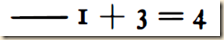is the True, whereas both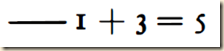and also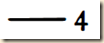are the False. Thus this function has as its value the argument itself, when that is a truth-value. I used to call this horizontal stroke the content-stroke (Inhaltsstrich) – a name that no longer seems to me appropriate. I now wish to call it simply the horizontal.

(34)

So consider if we write 5 > 4. Here we normally are expressing a judgment, namely in this case that 5 is grater than 4. [I am not sure, but it seems Frege is making the following distinction. Suppose using the notation before, we have a line with 5 > 4 to the right of it. This is something which is either true or false. But this is different if we were to assert that ‘5 > 4’ is true. This also would be assigned a truth value, but in this case it is the value of a judgment and not of a relation between terms. I probably understand this wrong, so consult the quotation to follow. At any rate, Frege will then add the vertical stroke, what he in the Begriffsschrift called the judgment stroke, and it seems to mean that not only are we stating the formulation but we furthermore are judging it to be true.]

If we write down an equation or inequality, e.g. 5 > 4, we ordinarily wish at the same time to express a judgment; in our example, we want to assert that 5 is greater than 4. According to the view I am here presenting, ‘5 > 4’ and ‘1 + 3 = 5’ just give us expressions for truth-values, without making any assertion. This separation of the act from the subject-matter of judgment seems to be indispensable; for otherwise we could not express a mere supposition – the putting of a case without a p. 22 J simultaneous judgment as to its arising or not. We thus need a special sign in order to be able to assert something. To this end I make use of a vertical stroke at the left end of the horizontal, so that, e.g., by writing

we assert that 2 + 3 equals 5. Thus here we are not just writing down a truth-value, as in

2 + 3 = 5,

but also at the same time saying that it is the True.

(Frege 34)

[His next function seems to be negation. The function’s value is true when the arguments are false and vise versa. He then uses the negation stroke and the affirmation stroke as having combination properties like mathematical positive and negative. He then gives some examples that are not hard to follow. The interesting one will be when he says that the negation of the function with just 2 in it is true, because the original one was false. But I am not sure I understand exactly what is meant by just having the stroke plus the 2 means.]

The next simplest function, we may say, is the one whose value is the False for just those arguments for which the value of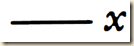is the True, and, conversely, is the True for the arguments for which the value ofis the False. I symbolize it thus: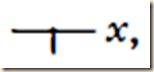| and here I call the little vertical stroke the stroke of negation. I conceive of this as a function with the argument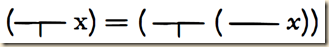where I imagine the two horizontal strokes to be fused together. But we also have: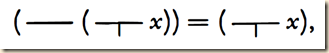since the value of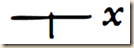is always a truth-value. I thus regard the bits of the stroke in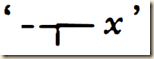to the right and to the left of the stroke of negation as horizontals, in the sense of the word that I defined previously. Accordingly, e.g.: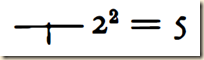stands for the True, and we may add the assertion-sign: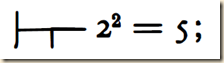and in this we assert that 22 = 5 is not the True, or that 22 is not 5. But moreover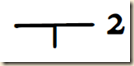is the True, since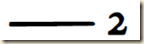is the False: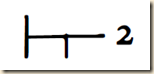i.e. 2 is not the True.

(34-35)

[Frege will now explain how to notate generality. He discusses this also in section 11 of his Begriffsschrift. The idea of ‘generality’ is universal quantification. The way it is notated is with a little indent to the line where the universally quantified variable is written, along with it being written with the function symbol to the right of the horizontal stroke. The scope is indicated by where the indent appears. In section 12 of Begriffsschrift he showed a number of combinations of forms for generality. If the negation stroke comes to the left of the universally quantified variable indent, then it is understood as something like “not all arguments make the function true”. And were the negation stroke to the right of the indent, then it would be understood as something like “all arguments make the negation of the function true.” If there is a negation stroke both before and after the indent, then it means something like “not all of the arguments make the negation of the function false” or put another way, “there is an argument that makes the function true.” In other words, we could designate existential quantification that way. This is a little confusing. Let me repeat the explanation I gave from section 12 of Begriffsschrift for this structure...

Suppose we have ‘for all x, x is not P.’ This means that none of the x’s are P. Now suppose we have ‘it is not the case that for all x, x is not P.” This would mean that we cannot say that none of the x’s are not P. It also would be making no claim whether or not all of them are either. In other words, it would seem to mean that some x’s are P.

... Or let me put it another way. If we say, not all the objects are lacking a property, that would mean some would have to have it.]

My way of presenting generality can best be seen in an example. Suppose what we have to express is that every object is equal to itself. In

x = x

we have a function, whose argument is indicated by ‘x.’ We now have to say that the value of this function is always the True, whatever we take as argument. I now take the sign

to mean the True when the function f(x) always has the True as its value, whatever the argument may be; in all other cases

p. 24]

| is to stand for the False. For our function x = x we get the first case. Thus

is the True; and we write this as follows:

The horizontal strokes to the right and to the left of the concavity are to be regarded as horizontals in our sense. Instead of ‘a,’ any other Gothic letter could be chosen; except those which are to serve as letters for a function, like f and F.

This notation affords the possibility of negating generality, as in

That is to say,

is the False, since not every argument makes the value of the function x2 = 1 to be the True. (Thus, e.g., we get 22 = 1 for the argument 2, and this is the False.) Now if

is the False, then

is the True, according to the rule that we laid down previously for the stroke of negation. Thus we have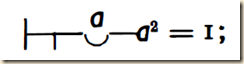i.e. ‘not every object is a square root of 1,’ or ‘there are objects that are not square roots of 1.’

p. 25] Can we also express: there are square roots of 1? Certainly: we need only take, instead of the function x2 = 1, the function

By fusing together the horizontals in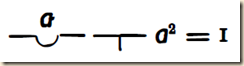we get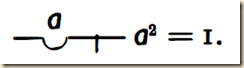| This stands for the False, since not every argument makes the value of the function

to be the True. E.g.: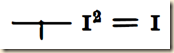is the False, for 12 = 1 is the True. Now since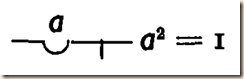is thus the False,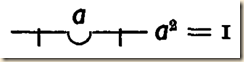is the True: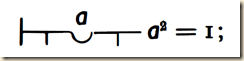i.e. ‘not every argument makes the value of the function

to be the True,’ or: ‘not every argument makes the value of the function x2 = 1 to be the False,’ or: ‘there is at least one square root of 1.’

(Frege 35-37)

Now with the structure for existential quantification, we can restate some formations into words. So if we say that there is a number that is greater to or equal to zero, then we are also saying there is at least one positive number: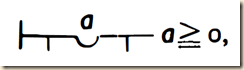(Frege 37)

[Here it seems that zero is counted as a positive number.] And thus we would write ‘there is at least one negative number’ as: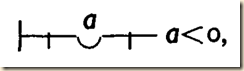[I do not grasp the next one. Let me just quote it.]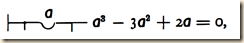there is at least one root of the equation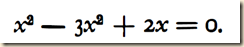(Frege 37).

Frege then emphasizes that this formation allows us to represent existential sentences. All the cases we saw are instantiations of the same form. If we render that form now using f as a function sign (as he puts it, of “an indefinite indication of a concept”), then we have: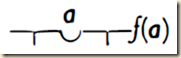[On the one hand we can think of the a as the argument. But were the function also variable, it could be seen as an argument, as in higher-order logics. See Nolt Logics section 14.1.] Frege says he also conceives

as the expression of a function whose argument is indicated by ‘f’. Such a function is obviously a fundamentally different one from those we have dealt with so far; for only a function can occur as its argument. Now just as functions are fundamentally different from objects, so also functions whose arguments are and must be functions are fundamentally different from functions whose arguments are objects and cannot be anything else. I call p. 27] the latter first-level, the former second-level, functions. In the same way, I distinguish between first-level and second-level concepts. Second-level functions have actually long been used in Analysis; e.g. definite integrals (if we regard the function to be integrated as the argument).

(Frege 38)

Frege turns now to the topic of functions taking two arguments. [Recall that a function is saturated when its argument is specified and unsaturated when it is not.] Frege first notes that “We get the expression for a function by splitting up the complex sign for an object into a ‘saturated’ and an ‘unsaturated’ part” (Frege 38). [Frege will begin with a “sign for the True”.  By this he is referring to a true function whose meaning is thus the True. The saturated part is the argument that fulfills the variable part of the function. And the unsaturated part is the remainder of the function where the variable part is given as a place-holder rather than specified as one argument or another. I am not certain about the next step, but he then seems to be saying that we can think of the unsaturated function itself as being variable, again a little like using higher-order logic to range over predicates themselves. (Again see Nolt Logics section 14.1.) Frege then makes a terminological distinction. When there is a function with just one argument, then it is called a concept. But when it has two arguments, we call it a relation. I am not sure why he selects the term ‘concept’ for a function with one argument. Is there something that is not conceptual about a relation? He then further clarifies what makes something a relation. The important feature seems to be that the value of one term has a determinative relation with respect to the other. So whatever one term is will constrain what the other one is. However, in expressions where there is no such constraining of the one on the other, it is not a relation. But please check the quotation to follow to be sure about this.]

I will now add something about functions with two arguments. We get the expression for a function by splitting up the complex sign for an object into a ‘saturated’ and an ‘unsaturated’ part. Thus, we split up this sign for the True,

3 > 2,

| into ‘3’ and ‘x > 2.’ We can further split up the ‘unsaturated’ part ‘x > 2’ in the same way, into ‘2’ and

x > y,

where ‘y’ enables us to recognize the empty place previously filled up by ‘2.’ In

x > y

we have a function with two arguments, one indicated by ‘x’ and the other by ‘y’; and in

3 > 2

p. 28] we have the value of this function for the arguments 3 and 2. We have here a function whose value is always a truth-value. We called such functions of one argument concepts; we call such functions of two arguments relations. Thus we have relations also, e.g., in

x2 + y2 = 9

and in

x2 + y2 > 9

and in whereas the function

x2 + y2

has numbers as values. We shall therefore not call this a relation.

(Frege 39)

[For the next idea, let us first recall from section 5 of his Begriffsschrift how Frege notates conditional propositions. Let me first show the structure: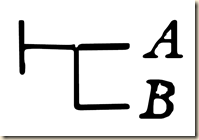Given the alphabetical order and conventions we might be accustomed to, we might be tempted to read this as “A→B”. But in fact, given how Frege defines the structure, it should be read as “B→A”. Here is his explanation:

If A and B stand for possible contents of judgment (§ 2), we have the four following possibilities:
(i) A affirmed, B affirmed;
(ii) A affirmed, B denied;
(iii) A denied, B affirmed;
(iv) A denied, B denied.stands for the judgment that the third possibility is not realized, but one of the other three is. (Begriffsschrift 5)

]

At this point I may introduce a function not peculiar to arithmetic. The value of the function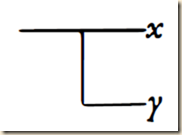is to be the False if we take the True as the y-argument and at the same time take some object that is not the True as the x-argument; in all other cases the value of this function is to be the True. The lower horizontal stroke, and the two bits that the upper one is split into by the vertical, are to be regarded as horizontals [in our sense]. Consequently, we can always regard as the arguments of our function–––x and–––y, i.e. truth-values.

(39)

[For the next idea, let us first recall the cases we dealt with before with one argument but some differed in that there were of a higher level. Those on the first level, it seems, are such because their function is specified. But when we make the function itself a variable, it then becomes second-level. I am not certain, but his point now seems to be that in these cases of one-argument functions, if it is second-level, then it is so entirely, and if it is first-level, then it is so entirely. I am not exactly sure why, but perhaps it has something to do with the structure not being composite. I am guessing. At any rate, he seems to be saying now that with the conditional structure we can mix functions of different orders. He also says that the differential quotient is also an unequal-leveled function. Here, he might be saying that we think of derivation of a function, and as its input is the original function, which could be a variable of a certain power, like f(x)=x2, and the derivative being of a lower power, like f ' (x) = 2x. Or perhaps he is saying that the original function is first-level, because the argument is variable but the function f is not. But the derivative f ' is itself a function whose argument is another function, which can be said to be variable, and this function itself has its own variable argument. I am just guessing, and I am less able to know what he means with the definite integral. The idea seems to be that we will find the definite integral for a function, and this function is first-level. Then the definite integral is a function takes the upper limit of the first function as its argument. Again I am guessing wildly. For more on the differential quotient, see David Jerison’s class here and Edward’s and Penney’s text here. For more on the definite integral see David Jerison’s class here and Edward’s and Penney’s text here. Frege then says that for equal-leveled functions, they are either first- or second-level. He then gives an example of a second-level one that seems to be a function applied to another function. For more on this structure, see Suppes Intro section 11.2.]

Among functions of one argument we distinguished first-level and second-level ones. Here, a greater multiplicity is possible. | A function of two arguments may be of the same level in relation p. 29] to them, or of different levels; there are equal-levelled and unequal-levelled functions. Those we have dealt with up to now were equal-levelled. An example of an unequal-levelled function is the differential quotient, if we take the arguments to be the function that is to be differentiated and the argument for which it is differentiated; or the definite integral, so long as we take as arguments the function to be integrated and the upper limit. Equal-levelled functions can again be divided into first-level and second-level ones. An example of a second-level one is

F(f(1)),

where ‘F’ and ‘f’ indicate the arguments.

(Frege 39-40)

[The next idea I am uncertain about, so you will need to read the quotation to follow. I will try to say some things about this section. Here we are dealing with second-level functions with one argument. So if it is second-level with one argument, that means it is a second-level function whose one argument is another function. Next we need to distinguish a second-level function with one argument where that one argument is a function with just one argument of its own and a second-level function with one argument where that one argument is a function with two arguments of its own. His next point seems to be the following. Suppose again we have a second-level function of one argument. Frege says that we cannot arbitrarily put as its argument another function either of one or alternately of two arguments. This is because one or the other creates a very different second-order function, and so they are not interchangeable. Thus we must distinguish the two kinds of second-level functions on the basis of how many arguments its argument takes. Frege then gives an example of a second-level function with one argument where that one argument itself takes two arguments of its own. I will show it here but it will repeat in the quotation below.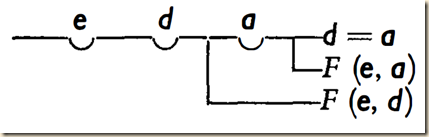I really do not know how to decipher this properly, but let us still try to say some things about it. He says that the function with two arguments is F, and its two arguments are e and a in one case and e and d in another case. But that would make it I think be the first-level function. So then, where is the second-level function? I am not sure how this works, but I would guess that the second-level function is implied in the fact that the first-level function is itself a variable F. So while there are no indentations in the horizontal lines which quantify for F, perhaps they are understood somehow to be implicitly in the structure. I again do not know how to decipher this formulation properly, but perhaps we might wonder if it is something similar to this structure here:

edF(Fed → ∀a(Fead=a))

]

In regard to second-level functions with one argument, we must make a distinction, according as the role of this argument can be played by a function of one or of two arguments; for a function of one argument is essentially so different from one with two arguments that the one function cannot occur as an argument in the same place as the other. Some second-level functions of one argument require that this should be a function with one argument; others, that it should be a function with two arguments; and these two classes are sharply divided.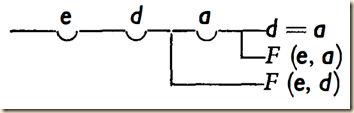p. 30] is an example of a second-level function with one argument, which requires that this should be a function of two arguments. The letter F here indicates the argument, and the two places, separated by a comma, within the brackets that follow ‘Fbring it to our notice that F represents a function with two arguments.

(Frege 40)

[And given the complexity here of second-level functions with one argument, we can imagine how structures can be even more elaborate when the second-level function takes two arguments.] “For functions of two arguments there arises a still greater multiplicity” (Frege 40).

Frege’s next points is that as arithmetic developed, it came to recognize and analyze structures beginning on the lowest order and eventually increasing to higher and higher order functions. One of Frege’s observations is that when people started using first-level functions, they did not have yet a concept of function. Then as people began to recognize functions and to formulate second-level ones, they still at first did not have a concept of higher-order functions. [Frege next might be saying that he himself has here first given conceptual formulation to the notion of a higher-order function, but I am not sure. Here he writes: “Now at this point people had particular second-level functions, but lacked the conception of what we have called second-level functions. By forming that, we make the next step forward.” In section 14.1 of Nolt’s Logics he says that Frege invented higher-order logic (Nolt 383). I do not follow Frege’s next point so well, but he emphasizes that the difference between first and second-level functions “is not made arbitrarily, but founded deep in the nature of things”. And his final point seems to be that there is a basic distinction between functions that have one argument and functions that have two arguments. But I am not sure why this would be the final point he makes in the presentation.]

If we look back from here over the development of arithmetic, we discern an advance from level to level. At first people did calculations with individual numbers, 1, 3 , etc.

2 + 3 = 5,  2.3 = 6

| are theorems of this sort. Then they went on to more general laws that hold good for all numbers. What corresponds to this in symbolism is the transition to the literal notation. A theorem of this sort is

(a + b)c = a.c + b.c.

At this stage they had got to the point of dealing with individual functions; but were not yet using the word, in its mathematical sense, and had not yet formed the conception of what it now stands for. The next higher level was the recognition of general laws about functions, accompanied by the coinage of the technical term ‘function.’ What corresponds to this in symbolism is the introduction of letters like f, F, to indicate functions indefinitely. A theorem of this sort is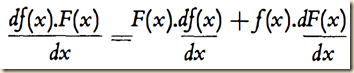p. 3 I] Now at this point people had particular second-level functions, but lacked the conception of what we have called second-level functions. By forming that, we make the next step forward. One might think that this would go on. But probably this last step is already not so rich in consequences as the earlier ones; for instead of second-level functions one can deal, in further advances, with first-level functions – as shall be shown elsewhere. But this does not banish from the world the difference between first-level and second-level functions; for it is not made arbitrarily, but founded deep in the nature of things.

Again, instead of functions of two arguments we can deal with functions of a single but complex argument; but the distinction between functions of one and of two arguments still holds in all its sharpness.

(Frege 41-42)

From:

Frege, Gottlob. “Function and Concept.” Transl. P.T. Geach. In Translations from the Philosophical Writings of Gottlob Frege. Eds. P.T. Geach and Max Black. pp. 21–41. Oxford: Basil Blackwell, 1960, second edition (1952 first edition).

.

Or if otherwise noted:

Frege, Gottlob. “Funktion und Begriff.” In Funktion, Begriff, Bedeutung. Fünf logische Studien. Edited by Günther Patzig. pp.1–22. Göttingen: Vandenhoeck & Ruprecht, 2008 .

Frege, Gottlob. “Begriffsschrift (Chapter 1)”. Transl. P.T. Geach. In Translations from the Philosophical Writings of Gottlob Frege. Eds. P.T. Geach and Max Black. Oxford: Basil Blackwell, 1960, second edition (1952 first edition).

Nolt, John. Logics. Belmont, CA: Wadsworth, 1997.